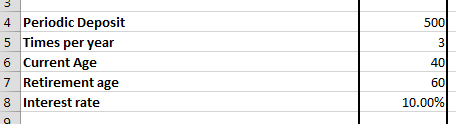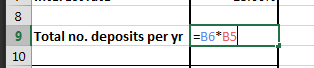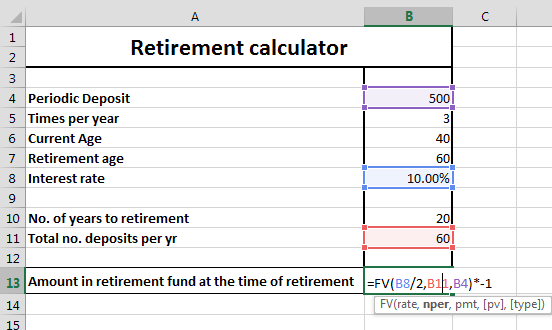### Retirement Calculator In Excel

Thanks to Excel you can easy calculate things. Here you have free retirement calculator to find out how much will get being senior.1. Open Excel and make four rows which are periodic deposit, times per year, current age, retirement age, interest rate, no. of years to retirement, and total no. of deposits per year.2. Enter your data in the cells corresponding the headings: periodic deposit, times per year, current age, retirement age, and interest rate.3. Calculate no. of years to retirement and total no. of deposits per year. No. of years to retirement is calculated by deducting current age from retirement age.4. Total no. of deposit is simply calculated by multiplying times per year and no. of years.5. Now calculate estimated savings using FV function in Excel in the cell corresponding estimated savings. Formula goes as follows:

=PV(interest rate/times per year, total number of deposits per year, periodic deposits)*-1The full formula of retirement calculator is:

=FV(B8/2,B11,B4)*-1

```Further reading: